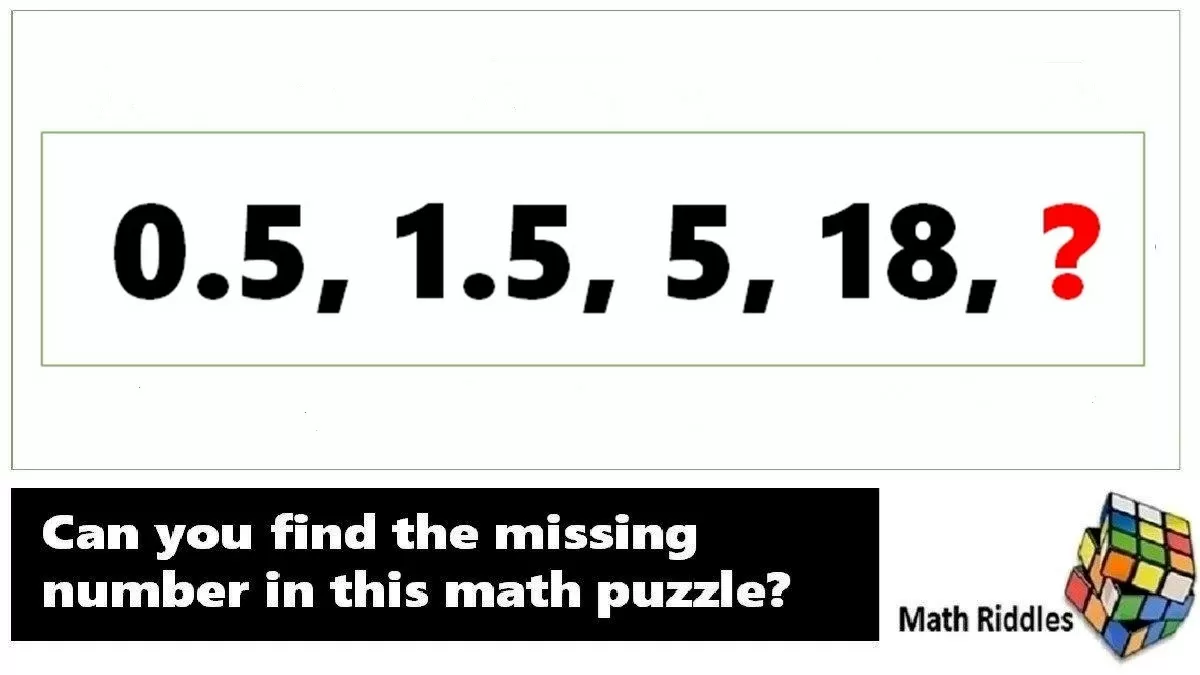# Math Riddles: Missing Number Series Puzzles, Solve in 20 Seconds Each

## Math Riddles Challenge: Can you find the missing numbers in these math puzzles? Check Your IQ.Math Riddles: Missing Number Series Puzzles, Solve in 20 Seconds Each

Math Riddles with Answers: Today, we will solve numerical reasoning problems. In these missing number series, you have to find the numbers in the place of the question marks. We bet you will find these puzzles hard to solve. Figure out the logic and try to solve each puzzle in 20 seconds. Let begin.

## Tell us in comments: Did you solve these math puzzles in 20 seconds each?

Check out more math puzzles!